HOTS Questions: Forces & Laws of Motion

# HOTS Questions: Forces & Laws of Motion - Class 9

Test Description

## 25 Questions MCQ Test Science Class 9 - HOTS Questions: Forces & Laws of Motion

HOTS Questions: Forces & Laws of Motion for Class 9 2023 is part of Science Class 9 preparation. The HOTS Questions: Forces & Laws of Motion questions and answers have been prepared according to the Class 9 exam syllabus.The HOTS Questions: Forces & Laws of Motion MCQs are made for Class 9 2023 Exam. Find important definitions, questions, notes, meanings, examples, exercises, MCQs and online tests for HOTS Questions: Forces & Laws of Motion below.
Solutions of HOTS Questions: Forces & Laws of Motion questions in English are available as part of our Science Class 9 for Class 9 & HOTS Questions: Forces & Laws of Motion solutions in Hindi for Science Class 9 course. Download more important topics, notes, lectures and mock test series for Class 9 Exam by signing up for free. Attempt HOTS Questions: Forces & Laws of Motion | 25 questions in 25 minutes | Mock test for Class 9 preparation | Free important questions MCQ to study Science Class 9 for Class 9 Exam | Download free PDF with solutions
 1 Crore+ students have signed up on EduRev. Have you?
HOTS Questions: Forces & Laws of Motion - Question 1

### Match the following with correct response.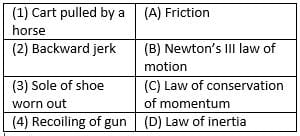Detailed Solution for HOTS Questions: Forces & Laws of Motion - Question 1

1) A cart is pulled by the horse as the horse applies a force on earth and earth applies a equal and opposite force. this is stated in Newton third law of motion.
2) We get a backward jerk due to inertia as the car speeds up and our body tends to move backwards and resist the change.
3) Sole of shoe worns out due to friction.
4) The spring in the gun transfers the momentum to the bulletand according to law of conservation of momentum the gun recoils.

HOTS Questions: Forces & Laws of Motion - Question 2

### Dust particles come out of a hanging carpet when we strike it with a stick because- A. Carpet starts moving forward and backward due to inertia of motion  B. Dust particles remain at rest  C. Dust particles starts moving forward due to inertia of motion D. Carpet remains at rest

Detailed Solution for HOTS Questions: Forces & Laws of Motion - Question 2
This is because when we hit a carpet the carpet starts moving forward and its inertia of rest changes into the inertia of motion but the dust particles which are there with the carpet they move just because of the carpet but actually the particles remain at rest that is why these particles fall of due to the change of inertia.
HOTS Questions: Forces & Laws of Motion - Question 3

### Find the incorrect statement about Newton’s law of action and reaction. Action and reaction

Detailed Solution for HOTS Questions: Forces & Laws of Motion - Question 3

Newton’s third law of motion explains action and reaction. Action and reaction acts simultaneously, are equal in magnitude. They act on different direction and acts on different body.

HOTS Questions: Forces & Laws of Motion - Question 4

If an object experiences a net zero unbalanced force, than the body

Detailed Solution for HOTS Questions: Forces & Laws of Motion - Question 4

If an object experience a net zero unbalanced force, than the body moves with constant velocity. Zero unbalanced force due to produce acceleration in the body.

HOTS Questions: Forces & Laws of Motion - Question 5

Match the following with correct response.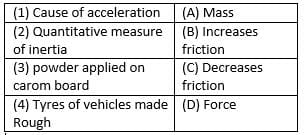Detailed Solution for HOTS Questions: Forces & Laws of Motion - Question 5

D is the correct option.Force is the cause of acceleration. Mass Is the quantitative measure Of inertia. We apply powder on the carrom board to decrease friction. Tyres of vehicles made rough to increase friction.

HOTS Questions: Forces & Laws of Motion - Question 6

A force of 7 kg-wt acts on a body of mass 10 kg calculate the acceleration produced.

Detailed Solution for HOTS Questions: Forces & Laws of Motion - Question 6

Force = 7 kg-wt = 7×10 = 70 N, mass = 10 kg. therefore, acceleration produced in the body = F/a = 70/10
= 7 ms-2.

HOTS Questions: Forces & Laws of Motion - Question 7

Which is incorrect statement about action and reaction referred to Newton’s third law of motion?
A. They are equal
B. They are opposite
C. They act on same object
D. They act simultaneously

Detailed Solution for HOTS Questions: Forces & Laws of Motion - Question 7

Newton’s third law states that for every action (force) in nature there is an equal and opposite reaction. In other words, if object A exerts a force on object B, then object B also exerts an equal and opposite force on object A.

For every action, there is an equal and opposite reaction. The statement means that in every interaction, there is a pair of forces acting on the two interacting objects. The size of the forces on the first object equals the size of the force on the second object.

The objects interact. The action and reaction force always act on different objects. Two forces acting on the same object, even if they have the same magnitude and point in opposite directions, never form an action-reaction pair.

HOTS Questions: Forces & Laws of Motion - Question 8

Statement A: A passenger falls forward when a bus suddenly starts moving in the forward direction
Statement B: A gun recoils backward with small speed than the bullet moving forward.

Detailed Solution for HOTS Questions: Forces & Laws of Motion - Question 8

A passenger falls backward when a bus suddenly start moving in the forward direction due inertia of rest. A gun recoils backward with small speed than the bullet moving forward due to law of conservation of momentum.

HOTS Questions: Forces & Laws of Motion - Question 9

When a force is exerted on an object, it can change its-
A. State
B. Position
C. Shape
D. Direction

Detailed Solution for HOTS Questions: Forces & Laws of Motion - Question 9

D is the correct option.A force acting on an object causes the object to change its shape or size, to start moving, to stop moving, to accelerate or decelerate. When there's the interaction between two objects they exert a force on each other, these exerted forces are equal in size but opposite in direction.

HOTS Questions: Forces & Laws of Motion - Question 10

The physical quantity which is equal to the rate of change of momentum is

Detailed Solution for HOTS Questions: Forces & Laws of Motion - Question 10

Force is the equal to the rate of change of momentum. Force = final momentum-initial momentum/time , f = mv-mu/t.

HOTS Questions: Forces & Laws of Motion - Question 11

Which of the following is not used to reduce friction

Detailed Solution for HOTS Questions: Forces & Laws of Motion - Question 11

To reduce the friction, oiling between contact surface, using ball bearing and using grease between contact surfaces. Making scratches between contact surfaces increase the friction.

HOTS Questions: Forces & Laws of Motion - Question 12

Match the following with correct response.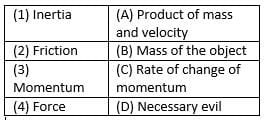HOTS Questions: Forces & Laws of Motion - Question 13

The instruments that make our work easier are called

Detailed Solution for HOTS Questions: Forces & Laws of Motion - Question 13
The instruments that make our work easier are called machines. Machines are tools or devices that are designed to perform specific tasks or functions. They are created to simplify and automate various processes, making work more efficient and less labor-intensive. Here are some key points to consider:
- Definition: Machines are mechanical or electrical devices that use energy to perform work. They can be simple, such as a lever or a pulley, or complex, like a computer or a car.
- Types: There are various types of machines, each designed for a specific purpose. Some common examples include generators, motors, and electric currents. However, it is important to note that these are specific types of machines, and the term "machine" itself is a more general term.
- Function: Machines are used to make work easier by reducing the amount of force, energy, or time required to complete a task. They can increase productivity, improve precision, and enable humans to perform tasks that would otherwise be impossible or extremely difficult.
- Examples: Machines can be found in almost every aspect of our daily lives. Some common examples include cars, computers, washing machines, elevators, and power tools. These machines have revolutionized industries and have greatly enhanced our quality of life.
- Importance: Machines have had a profound impact on society, driving technological advancements and shaping the way we live and work. They have played a crucial role in industrialization, automation, and the development of new industries.
In conclusion, machines are the instruments that make our work easier. They are designed to perform specific tasks or functions, using energy to simplify and automate various processes. From simple tools to complex devices, machines have revolutionized industries and greatly enhanced our quality of life.
HOTS Questions: Forces & Laws of Motion - Question 14

Which of the following physical quantity is associated with Newton’s second law of motion?

Detailed Solution for HOTS Questions: Forces & Laws of Motion - Question 14

Newton’s second law of motion states that rate of change in momentum is equal to force applied. Momentum is the product of mass and velocity. So, mass, velocity and time all are associated with Newton’s second law of motion.

HOTS Questions: Forces & Laws of Motion - Question 15

What is the momentum of an object of mass 1/2 m, moving with a velocity 2v?

Detailed Solution for HOTS Questions: Forces & Laws of Motion - Question 15

Momentum of a body is equal to product of mass and velocity. Momentum​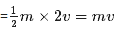HOTS Questions: Forces & Laws of Motion - Question 16

Match the following with correct response.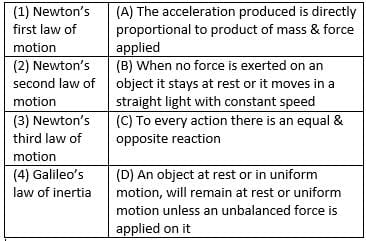HOTS Questions: Forces & Laws of Motion - Question 17

An Object moving along a straight path with an accelerated motion. The correct statement about the object-
A. Its velocity keeps changing
B. Its speed keeps changing
C. A force is always acting on it
D. Its mass keeps on changing

Detailed Solution for HOTS Questions: Forces & Laws of Motion - Question 17

The correct option is Option C.
This is because when acceleration increases so will speed and velocity. As acceleration is a type of accelerated force a force will always act on the body.

HOTS Questions: Forces & Laws of Motion - Question 18

State whether the following statement is true or false “force can be measured using first law of motion”

Detailed Solution for HOTS Questions: Forces & Laws of Motion - Question 18

The force can be measured by newton’s second law of motion. First law of motion states that object in rest or uniform motion tends to remain in its state.

HOTS Questions: Forces & Laws of Motion - Question 19

“Internal forces are forces which bodies exert on each other when the bodies are part of the system” is

Detailed Solution for HOTS Questions: Forces & Laws of Motion - Question 19

Internal forces are those forces which exert on each other when the bodies are part of the system. The forces acting between the atoms of molecules that keep them together are example of internal force.

HOTS Questions: Forces & Laws of Motion - Question 20

Match the following with correct response.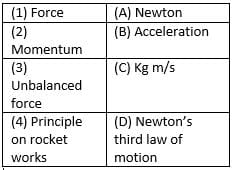HOTS Questions: Forces & Laws of Motion - Question 21

The passenger feels a jerk forward when a bus moving at a high speed suddenly stops. This happens due to

Detailed Solution for HOTS Questions: Forces & Laws of Motion - Question 21

When a moving bus at high speed suddenly stops, the passenger feels jerk in forwarding direction due to the inertia of motion, which tends to keep the moving body in motion.

HOTS Questions: Forces & Laws of Motion - Question 22

“A body moves with a constant speed of 5m/s. This shows that no unbalanced forces are acting on the body” is a

Detailed Solution for HOTS Questions: Forces & Laws of Motion - Question 22

If no unbalance force acts on the body, the speed of body remain constant. So, a body moving with constant speed of 5m/s does not have any unbalanced force acting on it.

HOTS Questions: Forces & Laws of Motion - Question 23

Match the following with correct response.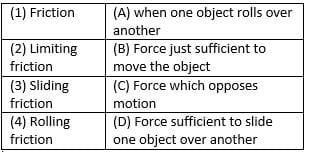HOTS Questions: Forces & Laws of Motion - Question 24

Weight of a body due to the Earth acts

Detailed Solution for HOTS Questions: Forces & Laws of Motion - Question 24

The weight of the body is the measure of force by which object is attracted towards the centre of earth. It always acts vertically downwards.

HOTS Questions: Forces & Laws of Motion - Question 25

Same amount of force is acting on a car and bus. It the ratio of the mass of the bus and the car is 50:1, calculate the ratio of the acceleration produced in the bus to the acceleration produced in the car

Detailed Solution for HOTS Questions: Forces & Laws of Motion - Question 25

F1= mass of car x acceleration produced in the car =m1*a1
F2= mass of bus x acceleration produced in the bus =m2*a2
m2/m1= 1:50
since F1= F2
m1*a1 =m2*a2
a1/a2 =m2/m
=1:50

## Science Class 9

66 videos|352 docs|97 tests
Information about HOTS Questions: Forces & Laws of Motion Page
In this test you can find the Exam questions for HOTS Questions: Forces & Laws of Motion solved & explained in the simplest way possible. Besides giving Questions and answers for HOTS Questions: Forces & Laws of Motion, EduRev gives you an ample number of Online tests for practice

## Science Class 9

66 videos|352 docs|97 tests